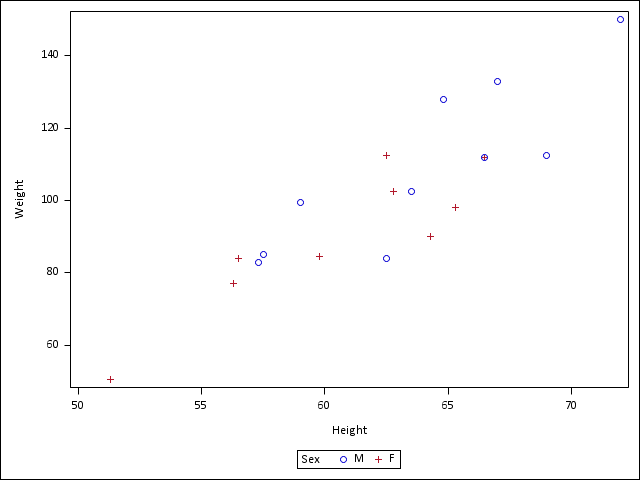## multilevel correlation matrix

Hi I need help plotting multilevel correlation in one plot. I want to plot correlation of speed vs. height for group 0,1, 2 and 3 all in one plot.

I tried the code below, but it gives me 3 separate plots.

``````proc corr data=mydata plots=matrix(nvar=all nwith=all);
VAR  height
with
Gaitspeed
;   BY group;
run; quit;``````

but I want just one plot with different color codes for the separate groups. any help much appreciated. thanks~

1 ACCEPTED SOLUTION

Accepted SolutionsPGStats
Opal | Level 21

## Re: multilevel correlation matrix

If you just want to plot the data, you will have more flexibility with plotting routines. For example:

``````proc sgplot data=sashelp.class;
scatter x=height y=weight / group=sex;
run;``````PG
2 REPLIES 2PGStats
Opal | Level 21

## Re: multilevel correlation matrix

If you just want to plot the data, you will have more flexibility with plotting routines. For example:

``````proc sgplot data=sashelp.class;
scatter x=height y=weight / group=sex;
run;``````PG

## Re: multilevel correlation matrix

perfect. thanks!

Discussion stats
• 2 replies
• 784 views
• 3 likes
• 2 in conversation